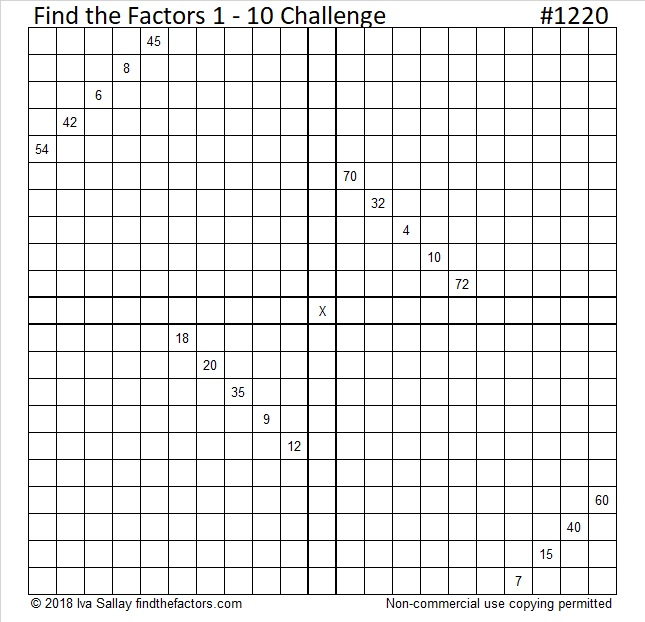1220 Challenge Puzzle

The last challenge puzzle was particularly difficult. This one won’t be nearly as bad. Try it and see if you can figure it out!Print the puzzles or type the solution in this excel file: 12 factors 1211-1220

Here are a few facts about the number 1220:

• 1220 is a composite number.
• Prime factorization: 1220 = 2 × 2 × 5 × 61, which can be written 1220 = 2² × 5 × 61
• The exponents in the prime factorization are 2, 1, and 1. Adding one to each and multiplying we get (2 + 1)(1 + 1)(1 + 1) = 3 × 2 × 2 = 12. Therefore 1220 has exactly 12 factors.
• Factors of 1220: 1, 2, 4, 5, 10, 20, 61, 122, 244, 305, 610, 1220
• Factor pairs: 1220 = 1 × 1220, 2 × 610, 4 × 305, 5 × 244, 10 × 122, or 20 × 61
• Taking the factor pair with the largest square number factor, we get √1220 = (√4)(√305) = 2√305 ≈ 34.92851220 is the sum of consecutive prime numbers: 607 + 613 = 1220

1220 is the sum of two squares two different ways:
32² + 14² = 1220
34² + 8² = 1220

1220 is the hypotenuse of four Pythagorean triples:
220-1200-1220 which is 20 times (11-60-61)
544-1092-1220 calculated from 2(34)( 8), 34² – 8², 34² + 8²
and is also 4 times (136-273-305)
828-896-1220 calculated from 32² – 14², 2(32)(14), 32² + 14²
and is also 4 times (207-224-305)
732-976-1220 which is (3-4-5) times 244